# Tableau Tip Tuesday: Don’t Use Table Calcs

No, not really!  I love table calculations! They are powerful and very useful.  They are one of the best things in Tableau.  Don’t be afraid of them and don’t avoid them, except…

• Avoid table calculations when a simpler aggregate calculation will work.
• Avoid aggregate calculations when a simpler row level calculation will work.

Why?  Because you lose control, flexibility, and efficiency.  When I first started to get the hang of table calcs, I found myself using them to solve every problem.  But that’s not the best approach.  The bottom line for this tip: keep it simple!“Your table calc complexity will only grow. There is beauty in it, but also great danger.”

# Row Level Instead of Aggregate

If you use an aggregate calculation, when you could have used a row level, then you will never* be able to use row level logic on that calculation.  Additionally, aggregate calculations must be computed at run-time.  Row level calculations may be materialized in an extract, meaning that they are only computed once.

*the only exception is when you use a Set or Level of Detail calc

Example:
You want the cost of goods sold when you have sales and profit.

Don’t do this: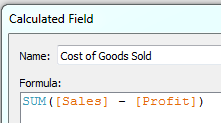You won’t be able to change it to an average cost of goods sold or compare a minimum and a maximum in the same view.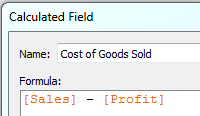As a row-level calculation, you’ll be able to specify the aggregation in the view.  It might also get materialized (computed once and stored) in an extract which means better efficiency.

Exception: When you specifically want to limit a designer using a published connection or web editing to a specific level of aggregation (e.g. you always want the designer to use average Cost of Goods Sold.)

# Aggregate (or Row Level) Instead of Table Calculation

If you use a table calculation when you could have used a simple aggregate, then you introduced a level of complexity that was not needed and made it harder for others to understand.  Furthermore, table calculations are calculated based on field arrangement in a view. So any changes to the level of detail or layout of the view can fundamentally change the way they work.  Simple aggregates are done at the level of detail defined by the dimensions in the view, but the fundamental calculation remains unchanged when you rearrange, add, or remove dimensions.

Example:
You want to add a reference line for the SUM of Sales for Appliances across the table to give a visual benchmark.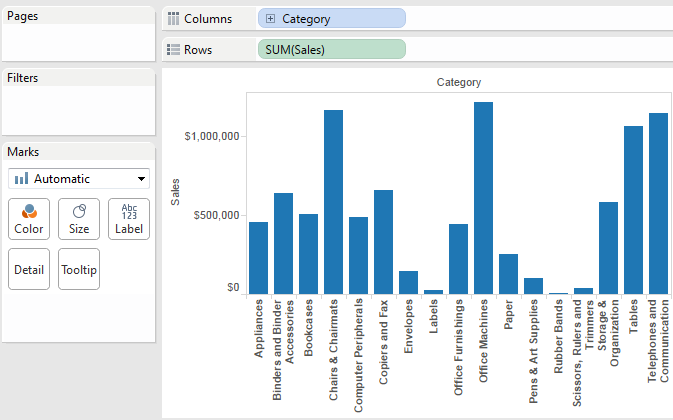Don’t do this: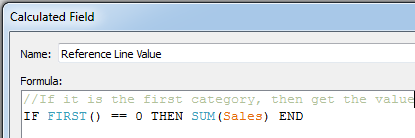Even if you can make the assumption that Appliances will always be first in terms of Category, how can you be certain that it will always be first based on the scope and direction of the First() table calculation when other dimensions are introduced?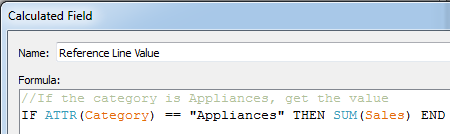As a simple aggregate, the calculation is less brittle and can be performed at the data source level.

Or, do this: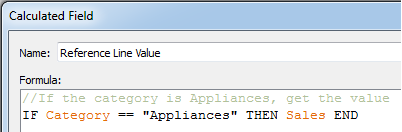Each row will keep the value for sales if it was an “Appliances” row (and NULL by default otherwise).  As a row level calculation, the results could potentially be materialized in an extract and is the most flexible.  You can choose to aggregate later.  And you can choose how.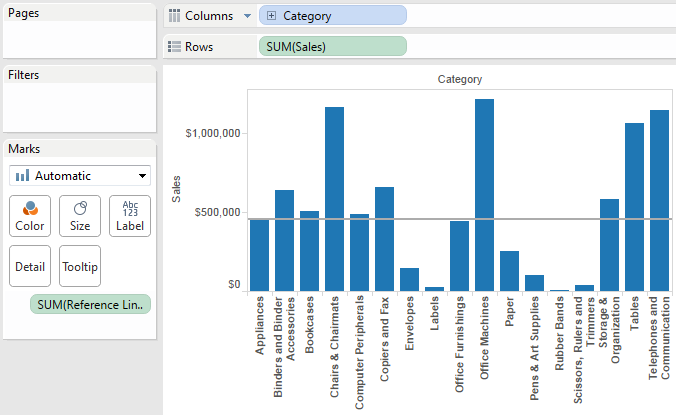When creating calculated fields, always look for the simplest approach.  Check back next Tuesday for another Tableau Tip!

### 4 Responses so far.

1.Arshad Malik says:

Excellent explanation, please guide how to learn Tableau and particularly Calculations.

Recommendations would be highly appreciated.

Regards

2.Lars says:

Good article.

But How do you calculate profit margin at row level? As I understood you get margin at each row but have to aggregate profit and aggregate Sales before using them in a division?

•benmelek_admin says:

Lars, great question!

So, this is a case where you wouldn’t want to calculate using a row level calculation because, as you point out, you have to do the aggregations first. You’d want SUM([Profit]) / SUM([Sales]) instead of [Profit] / [Sales]. The advise to use a row-level calc if you can doesn’t mean you always can (and get meaningful results).

3. […] difficulty with table calculations is 1) you have to set the right addressing and partitioning (not too bad in this case) and 2) you […]